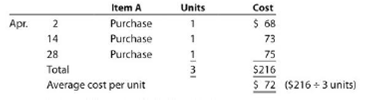Chapter 7, Problem 7.1APE

Chapter
Section
Textbook Problem

Cost flow methodsThe following three identical units of Item A are purchased during April:Assume that one unit is sold on April 30 for \$118.Determine the gross profit for April and ending inventory on April 30 using the (a) first-in, first-out (FIFO); (b) last-in, first-out (LIFO); and (c) weighted average cost methods.

(a)

To determine

Cost flow assumptions:

Cost flow assumptions can be referred to as the method which determines the flow of costs when an inventory is sold. Instead of keeping a track record for cost of each product sold, a company adopts these methods, and identifies which units were sold. It differs from specific identification method because the actual physical movement of goods may be unrelated to the flow of costs.

To determine: Gross profit and ending inventory under First-in-first out (FIFO) method.

Explanation

Here the first units purchased are expected to be sold first. Therefore, April 2 units are assumed to be sold...

(b)

To determine
Gross profit and ending inventory under Last-in-first out (LIFO) method.

(c)

To determine
Gross profit and ending inventory under weighted average inventory method.

Still sussing out bartleby?

Check out a sample textbook solution.

See a sample solution

The Solution to Your Study Problems

Bartleby provides explanations to thousands of textbook problems written by our experts, many with advanced degrees!

Get Started

Find more solutions based on key concepts